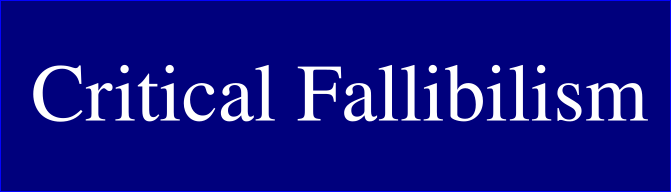# Justin Does Math

me2

Let S = black square, T = small triangle, and P = trapezoid

Original square: 400 = 1S + 4T + 4P
New Square: 400 - (T + P) = 1S + 3T + 3P

I’m not sure how to do a useful algebraic manipulation here. It seems like I need more info.

You can maybe see why you need more info from that formula, which would give you ideas about what to look for. Or just find more things and write them down.

ok

Did you find any other squares?

well there are three others similar to that first one (this image might be a little unclear but hopefully u get the idea)

There are also rectangles e.g.:

Oh hmm.

So this one has 1S + 5T + 5P.
It also is made up of 6 little squares, visually.
The smaller red square I outlined above was 1S + 3T + 3P and made up of 4 little squares, visually.
Looking at the picture, it looks like 1T + 1P = 1 square, and this is consistent with that (we have 2T + 2P more in the big rectangle vs the red-outlined square, and we have two more little squares in the picture).

New rectangle: 1S + 5T + 5P = 400 + (T + P)

I’m going through the thread so far and organizing the information in the form of a draft blog post to see if that helps.

notes or tree would be better

Figured out something else:

(note that the blue triangle is supposed to be within the purple triangle)

The big purple triangle has an area of 400. It’s composed of 1S + 4T + 4P. So 1S + 4T + 4P = 400. We knew that already.

The little blue triangle has area 100. It’s composed of 2T + 1P. So 2T + 1P = 100.

If we cut the blue triangle out of the purple triangle, we have a purple “band” where 1S + 2T + 3P = 300.

That’s a good thing to notice, but unfortunately, I think that one is not going to turn out mathematically convenient to get an answer from.

I think your main problem is some kind of suppression of brainstorming.

But if the question in the book was to find every square in my pic, of any size, and count them, I think you could do it. And then write down a formula for each different type of square.

Ah doh.

That sounds plausible.

I looked it up, and my assumptions about their intended solution were wrong, btw.

What they do is closer to what Elliot did than what I did.

Hmm this square seems important (writing more)

I think there’s something a bit weird about what you do & do not block. I’m not really sure what’s going on.

I think there are things you know that you didn’t list in brainstorming.

And you are doing things like not using pythagorean theorem, because you think that’s not part of the intended solution, but you are using equations in a way that looks like it could be leading to systems of equations. But I don’t think those would be part of the intended solution either (I think it’s a pre-algebra book).

One thing you could do is try to solve it any way you know how, including with pythagorean theorem, and then go back and try to find other ways to solve it too.

With your brainstorming, you could try to write down everything you can figure out about it, without actually trying to put it all together, and then go back through & look at that. I don’t think you ever did a step like that.

1 Like

I actually there may actually be an important point here.

I think something like this may be happening: you are not allowing yourself to use pythagorean theorem because you think the book didn’t intend pythagorean theorem to be used. So you want to solve it the way the book intended. You think that the students reading the book could solve it without using pythagorean, so you should be able to too.

Are you doing something like that?

(I have more to say, I just wanted to check if this is happening before I write more.)

1 Like

The square in the previous post has the formula 4T + 4P. If we relate it to one of the other squares with the black square, like the one in my earlier example, we could say 4T + 4P = 1S + 3T + 3P. That simplifies to 1T + 1P = 1S. We can connect this to the information from the original square (that 400 = 1S + 4T + 4P and get 5S = 400 and S = 80. So the shaded area is 80.

You’re still avoiding anything resembling brainstorming. How many squares are in my pic, and how many different types are there? (Or don’t answer but introspect about why you won’t do this or something.)

I started writing a reply to your earlier question about identifying the squares but then noticed the center square and wanted to follow my thinking about it - like i got excited about that thought or something. I’m going to answer ingracke’s question then come back to thinking about the squares.